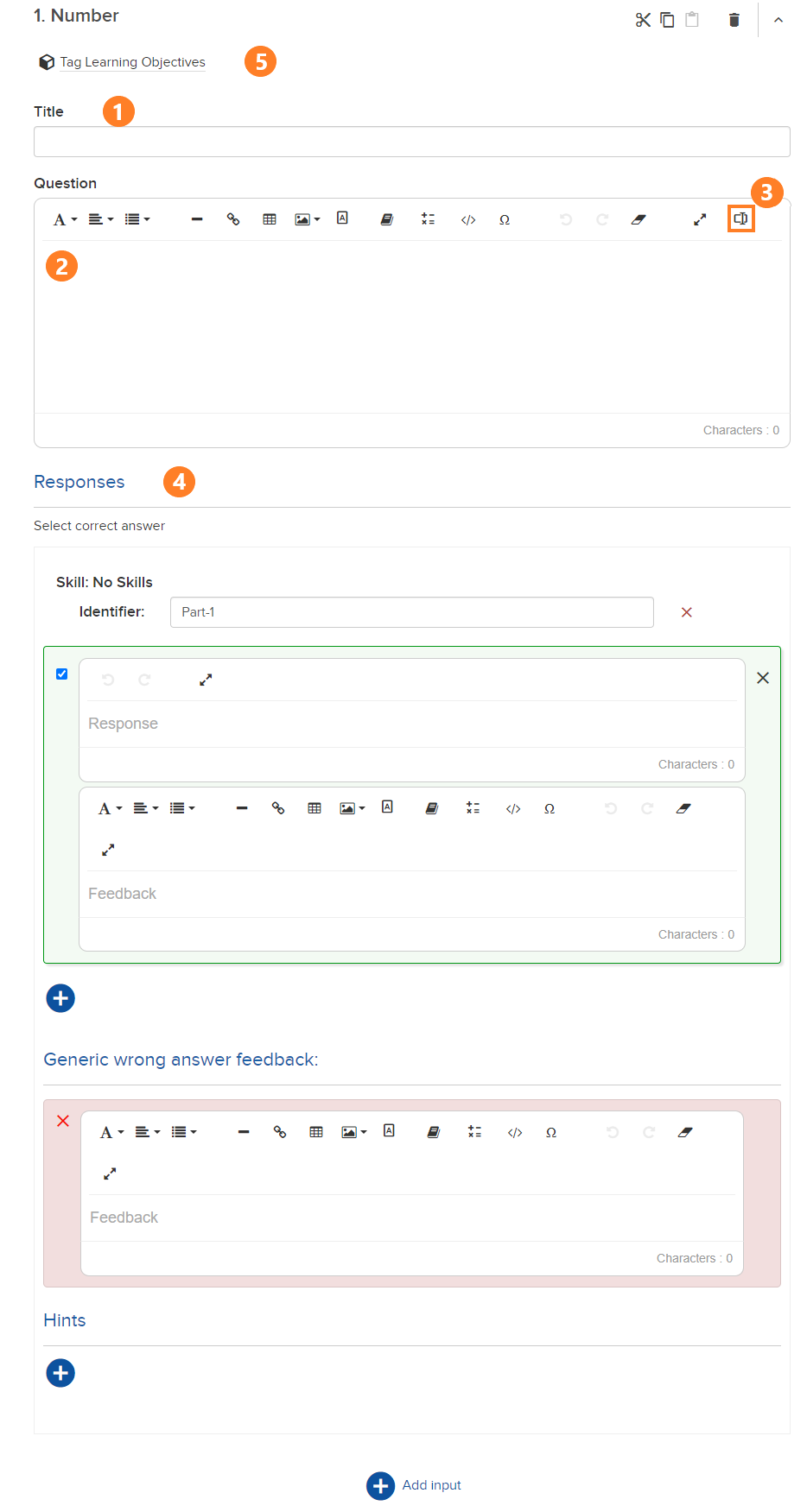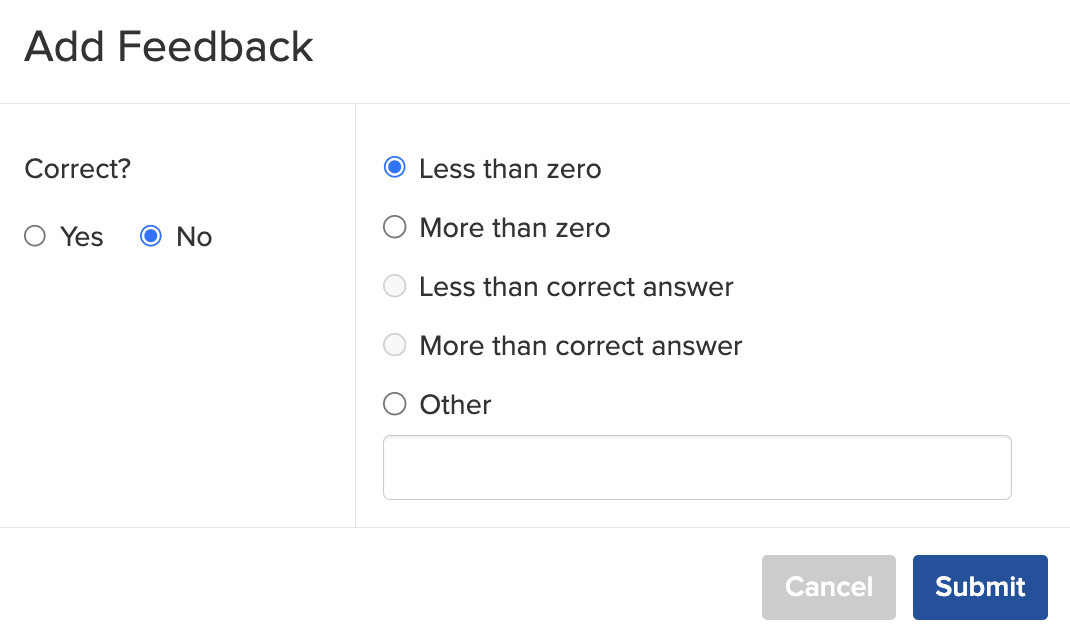# Number Input

In a Number Input question, students enter a number in a field. An answer designated as "correct" in Smart Author should include only digits and decimals. The student's answer must match the correct answer precisely, or you may enter multiple acceptable correct answers to accommodate differently formatted but equal numbers. You can also designate a range of answers.

Equivalent answers using 0 are also accepted. For instance, 0.001 is identical in value to .0010. However, it is best practice to provide directions to students as to how many decimal places they should enter.

• As an example, 0.001 is identical in value to .0010. Offer clear instruction on the expected format of the answer. Suggested language: "If the answer is between zero and one, be sure to include the zero to the left of the decimal point" or "Round to three decimal places."
• A range may be entered for the correct answer. For example, if you enter "[5,8]" the answers between 5 and 8 are marked correct or incorrect, however you map them.  Parentheses are exclusive ranges, so (5,8] excludes the number 5 but includes 8, as is standard in mathematical notation.
• If you wish an exact number to be accepted, be sure to use a 0 (zero) in the answer. For example, if you wish the answer to be 12, enter 12.0. If you do not, then 11.6 - 12.4 would be accepted as correct answers. If you wish the exact answer to be .8, enter .80 as the answer.

### Build a Number Input Question

Click + New Assessment, choose the assignment type, name the assessment, click Add, click + Add Content or Question, click + Add Question, and then click Number Input.

3. Insert Number Input using the icon indicated below.

5. Tag question.

###To add additional correct or specific incorrect answers and feedback, click the "+" below the responses. Doing so brings up the Add Feedback box.